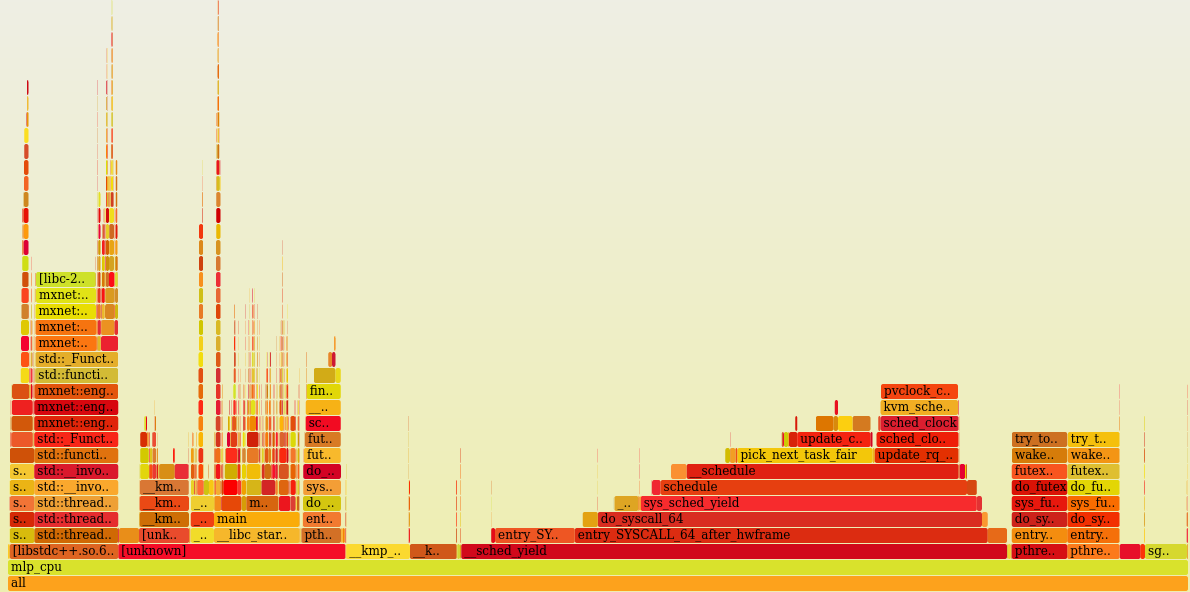# Performance Analysis of Deep Learning with Flamegraphs

In an earlier post, I had described a couple of ways you could gain insight into debugging C++ applications. There are several good tools to do something similar or better. However, this is a method I prefer using since `perf` is ubiquitous and easy to work with.

In this post, I simply want to explore whether it is possible to debug and profile Deep Learning Models in the same way that we generate `perf stat` and other profiling info for the models. We will take a relatively simple example and walk through it until we can get meaningful info/statistics from it.

MXNet is an open source Deep Learning library that is flexible and easy to understand. Real World DL Models can get very complex very fast with many hidden layers through which data is passed and compute heavy mathematical functions which are called often. These operators require significant optimizations or efficient implementations in the library.

### Multi-Layer Perceptron

Below we will look at a sample code for creating a Network with multiple layers that are full connected between each successive layers. The Activation function at each layer is `Relu` and the final one is a `softmax output`. It can be found under the examples section.

### Setup

#### Deep Learning Framework: MXNet

MXNet provides support for multiple languages through it’s APIs. `Python` is the lingua franca of the Deep Learning world, but it can be a little cumbersome to use as a target language to obtain profiler information from. So I decided to the `C++` API for this exercise. I compiled MXNet for the `C++` interface which needs to be built separately from source. `Intel's MKLDNN` Math Library was built from source and installed on the machine. In addition, MXNet was explicitly pointed to use this in our case.

After building this, you get a binary that is executable like any other C++ application since the model is now linked to the MXNet’s version under C++.

#### Training Data: MNIST Data:

Since the samples mostly work with MNIST Dataset, we will download it and use it for training. http://data.mxnet.io/mxnet/data/mnist.zip

``````Symbol mlp(const std::vector<int> &layers) {
auto x = Symbol::Variable("X");
auto label = Symbol::Variable("label");

std::vector<Symbol> weights(layers.size());
std::vector<Symbol> biases(layers.size());
std::vector<Symbol> outputs(layers.size());

for (size_t i = 0; i < layers.size(); ++i) {
weights[i] = Symbol::Variable("w" + std::to_string(i));
biases[i] = Symbol::Variable("b" + std::to_string(i));
Symbol fc = FullyConnected(
i == 0? x : outputs[i-1],  // data
weights[i],
biases[i],
layers[i]);
outputs[i] = i == layers.size()-1 ? fc : Activation(fc, ActivationActType::kRelu);
}

return SoftmaxOutput(outputs.back(), label);
}
``````

### Training Loop:

The training loop is simple enough to understand from the code and comments.

``````    for (int iter = 0; iter < max_epoch; ++iter) {
int samples = 0;
train_iter.Reset();

// train_iter is a MNIST Data Set Iterator
auto tic = std::chrono::system_clock::now();
while (train_iter.Next()) {
samples += batch_size;
auto data_batch = train_iter.GetDataBatch();
// Set data and label
data_batch.data.CopyTo(&args["X"]);
data_batch.label.CopyTo(&args["label"]);

exec->Forward(true);
exec->Backward();
// Update parameters
for (size_t i = 0; i < arg_names.size(); ++i) {
if (arg_names[i] == "X" || arg_names[i] == "label") continue;
}
}
auto toc = std::chrono::system_clock::now();

Accuracy acc;
val_iter.Reset();
while (val_iter.Next()) {
auto data_batch = val_iter.GetDataBatch();
data_batch.data.CopyTo(&args["X"]);
data_batch.label.CopyTo(&args["label"]);
// Forward pass is enough as no gradient is needed when evaluating
exec->Forward(false);
acc.Update(data_batch.label, exec->outputs);
}
float duration = std::chrono::duration_cast<std::chrono::milliseconds>
(toc - tic).count() / 1000.0;
LG << "Epoch: " << iter << " " << samples/duration << " samples/sec Accuracy: " << acc.Get();
}
``````

### Perf Output and Flamegraph:

Here it is.Flame Graphs for a Multi Layer Perceptron

A huge amount of time is spent in Data Iterator or handling page faults resulting in using the data iterator. `MKLDNN` makes things much more efficient. Almost all of them end up in `jit_avx32_gemm_`. The data loader causes the thread to yield. The other important part of the Control Flow Graph where a lot of time is spent is `Threads`

#### My Podcast!

If you like topics such as this then please consider subscribing to my podcast. I talk to some of the stalwarts in tech and ask them what their favorite productivity hacks are:

##### Available on iTunes Podcast